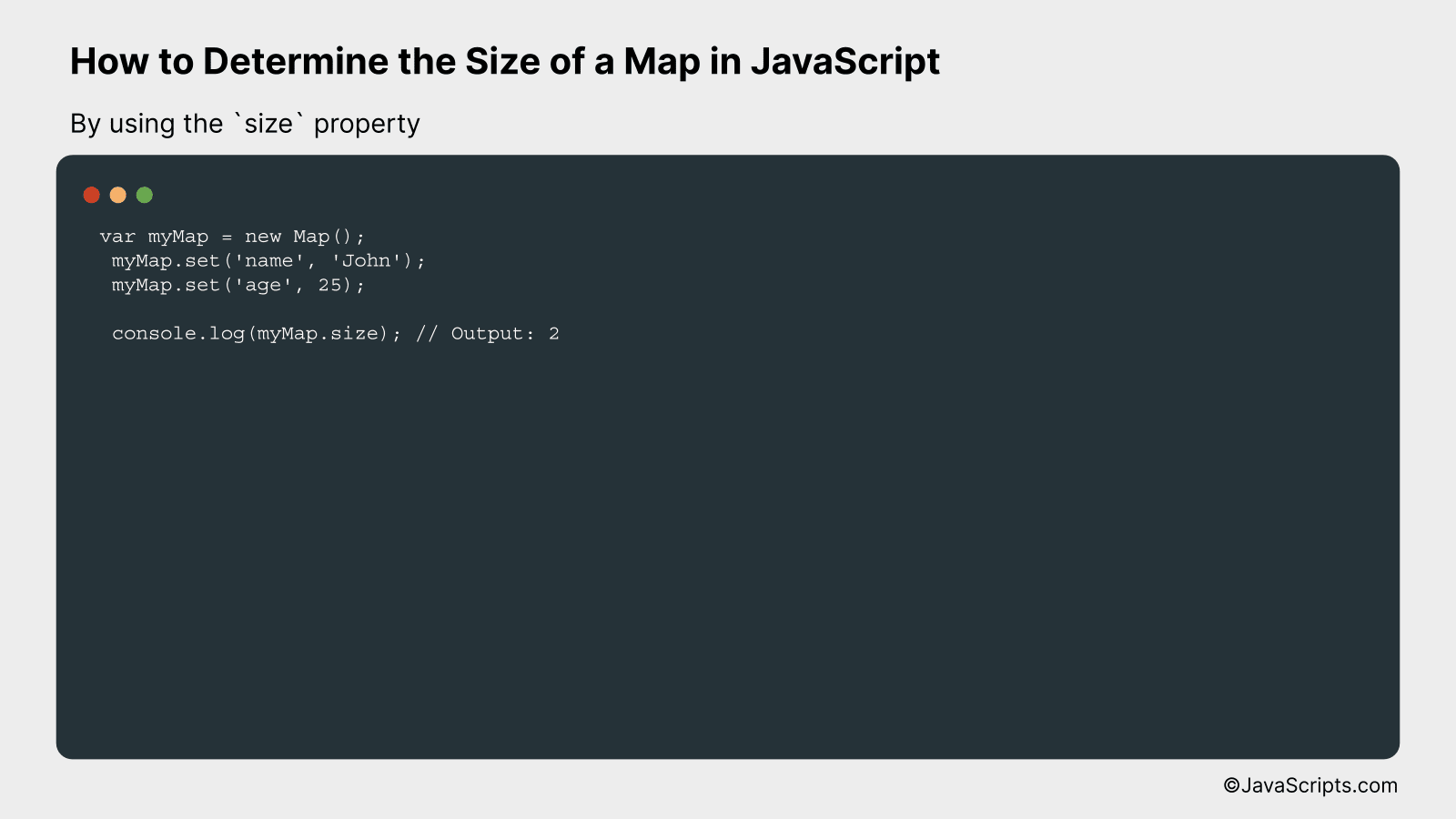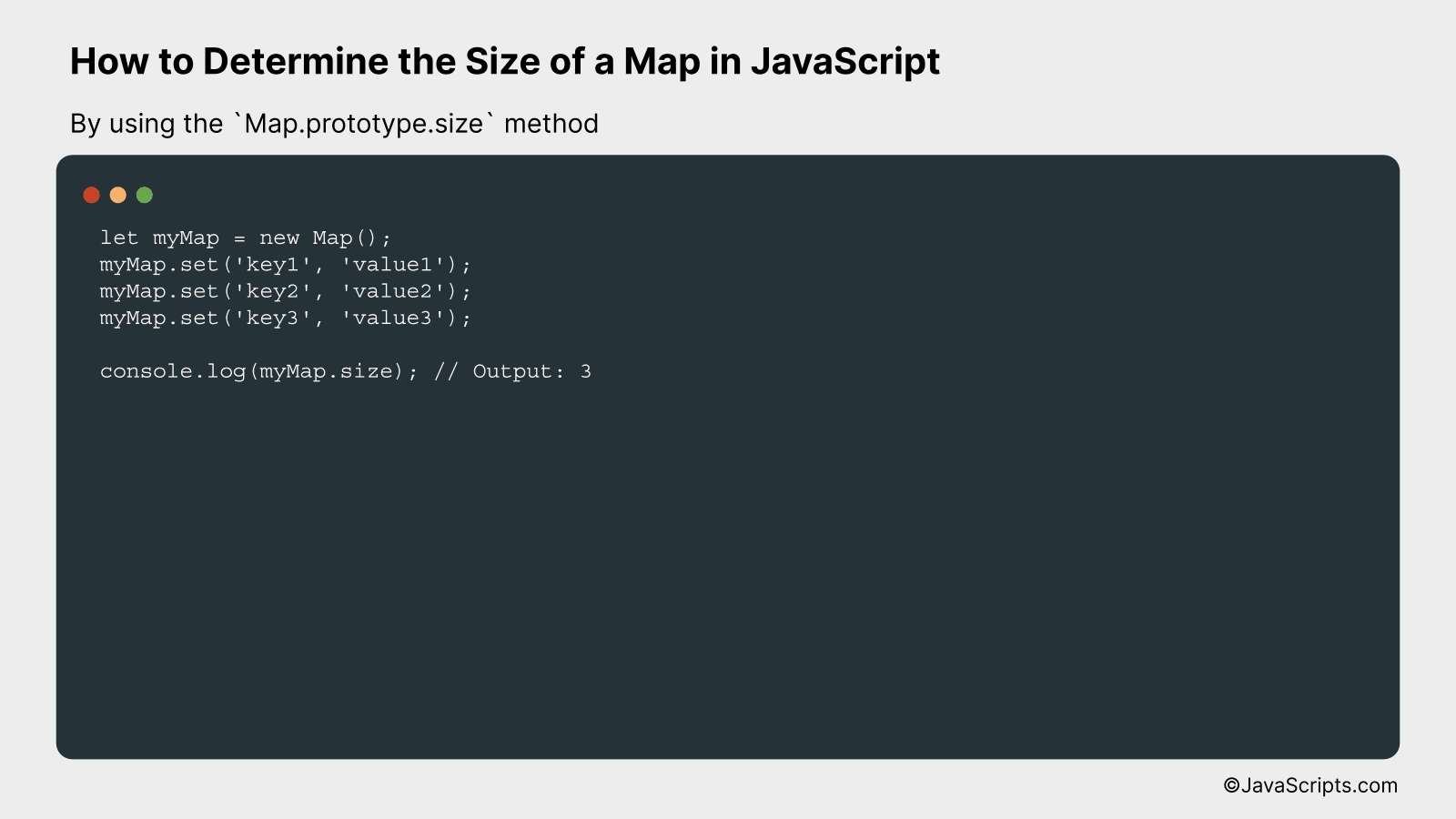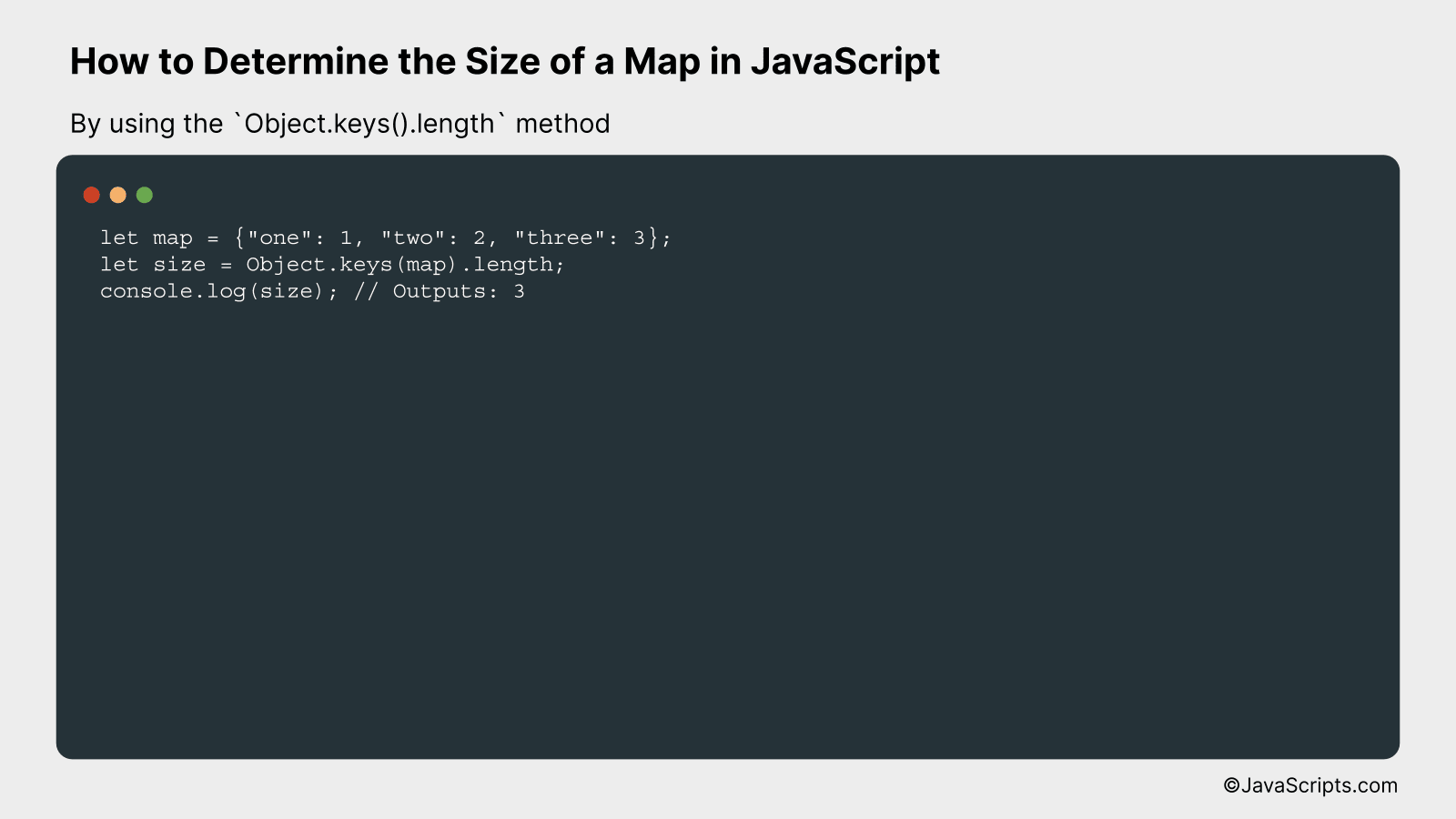# How to Determine the Size of a Map in JavaScript

You’re navigating the world of JavaScript and stumble upon a hurdle – how do you determine the size of a map? Don’t fret, because it’s something we can conquer together. Understanding the size of a map is crucial when dealing with data structures, as it directly impacts the performance and efficiency of your code.

Instead of being overwhelmed by abstract concepts, we’ll break this down into digestible pieces. We’re going to use an approachable, simple language that makes the process less intimidating and more achievable. Let’s dive into the intriguing world of JavaScript maps, together.

In this post, we will be looking at the following 3 ways to determine the size of a map in JavaScript:

• By using the size property
• By using the Map.prototype.size method
• By using the Object.keys().length method

Let’s explore each…

## #1 – By using the size propertyThe size property in JavaScript allows us to determine the number of elements in a Map object at any given moment. It’s a read-only property that returns the number of entries (key-value pairs) in the Map object. Here’s an example to illustrate how it works:

``````
var myMap = new Map();
myMap.set('name', 'John');
myMap.set('age', 25);

console.log(myMap.size); // Output: 2
``````

### How it works

The size property counts the number of entries in the Map object, and this count can change as entries are added or removed from the map.

• A new Map object, myMap, is created.
• Two entries are added to the myMap object using the set method; each entry consisting of a key (‘name’, ‘age’) and a corresponding value (‘John’, 25).
• When we call myMap.size, it returns the number of entries in the Map, which is 2 in this case.
• If we were to add or remove entries, the value returned by myMap.size would change accordingly.

## #2 – By using the Map.prototype.size methodThe Map.prototype.size property in JavaScript returns the number of key/value pairs present in a Map object. To understand this better, let’s consider an example where we create a Map, add some key-value pairs to it and use the ‘size’ property to determine the size of the map.

``````
let myMap = new Map();
myMap.set('key1', 'value1');
myMap.set('key2', 'value2');
myMap.set('key3', 'value3');

console.log(myMap.size); // Output: 3
``````

### How it works

The Map.prototype.size property works by simply counting the number of key-value pairs existing in the map. It requires no arguments, and it’s a read-only property.

• We begin by creating a new instance of a Map object using let myMap = new Map();
• Then, we add three key-value pairs to our map using the myMap.set(key, value) method.
• Finally, to get the number of key-value pairs or the size of the map, we use myMap.size. This is directly logged to the console in our example.
• The console logs 3, which is the number of key-value pairs in our map.

## #3 – By using the Object.keys().length methodThe Object.keys().length method in JavaScript is used to determine the size of a map by generating an array of the map’s keys with Object.keys() and then retrieving the length of that array with .length. This effectively gives you the number of key-value pairs in the map. Let’s illustrate this with an example.

``````
let map = {"one": 1, "two": 2, "three": 3};
let size = Object.keys(map).length;
console.log(size); // Outputs: 3
``````

### How it works

The Object.keys() method returns an array of a given object’s own enumerable property names, in the same order as they are obtained by a simple loop over the properties. When you call .length on that array, it returns the number of these property names, effectively giving the number of key-value pairs in the map.

• The variable map is initialized with a map containing three key-value pairs.
• Object.keys(map) is called, returning an array of the keys in map – in this case, [“one”, “two”, “three”].
• The .length property of the array is accessed, which gives the number of elements in the array – in this case, 3.
• This number is stored in the variable size and logged to the console, giving an output of 3 – the number of key-value pairs in map.

Related:

In conclusion, understanding the size of a map in JavaScript is an easy task. With the help of the ‘size’ property, we can determine it in no time. This property quickly counts the map elements for us.

Mastering this skill boosts your coding efficiency. With practice, you’ll find it more straightforward to manipulate data structures in JavaScript. Practice regularly, and soon you’ll find it a breeze!